Quantum Numbers and Electron Configurations

Quantum Numbers

The Bohr model was a one-dimensional model that used one quantum number to describe the distribution of electrons in the atom. The only information that was important was the size of the orbit, which was described by the n quantum number. Schrödinger's model allowed the electron to occupy three-dimensional space. It therefore required three coordinates, or three quantum numbers, to describe the orbitals in which electrons can be found.

The three coordinates that come from Schrödinger's wave equations are the principal (n), angular (l), and magnetic (m) quantum numbers. These quantum numbers describe the size, shape, and orientation in space of the orbitals on an atom.

The principal quantum number (n) describes the size of the orbital. Orbitals for which n = 2 are larger than those for which n = 1, for example. Because they have opposite electrical charges, electrons are attracted to the nucleus of the atom. Energy must therefore be absorbed to excite an electron from an orbital in which the electron is close to the nucleus (n = 1) into an orbital in which it is further from the nucleus (n = 2). The principal quantum number therefore indirectly describes the energy of an orbital.

The angular quantum number (l) describes the shape of the orbital. Orbitals have shapes that are best described as spherical (l = 0), polar (l = 1), or cloverleaf (l = 2). They can even take on more complex shapes as the value of the angular quantum number becomes larger.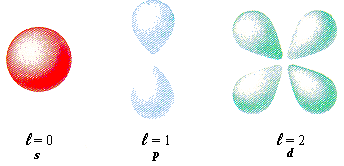There is only one way in which a sphere (l = 0) can be oriented in space. Orbitals that have polar (l = 1) or cloverleaf (l = 2) shapes, however, can point in different directions. We therefore need a third quantum number, known as the magnetic quantum number (m), to describe the orientation in space of a particular orbital. (It is called the magnetic quantum number because the effect of different orientations of orbitals was first observed in the presence of a magnetic field.)Rules Governing the Allowed Combinations of Quantum Numbers

• The three quantum numbers (n, l, and m) that describe an orbital are integers: 0, 1, 2, 3, and so on.
• The principal quantum number (n) cannot be zero. The allowed values of n are therefore 1, 2, 3, 4, and so on.
• The angular quantum number (l) can be any integer between 0 and n - 1. If n = 3, for example, l can be either 0, 1, or 2.
• The magnetic quantum number (m) can be any integer between -l and +l. If l = 2, m can be either -2, -1, 0, +1, or +2.

 Practice Problem 7:Describe the allowed combinations of the n, l, and m quantum numbers when n = 3. Click here to check your answer to Practice Problem 7Shells and Subshells of Orbitals

Orbitals that have the same value of the principal quantum number form a shell. Orbitals within a shell are divided into subshells that have the same value of the angular quantum number. Chemists describe the shell and subshell in which an orbital belongs with a two-character code such as 2p or 4f. The first character indicates the shell (n = 2 or n = 4). The second character identifies the subshell. By convention, the following lowercase letters are used to indicate different subshells.

 s: l = 0 p: l = 1 d: l = 2 f: l = 3

Although there is no pattern in the first four letters (s, p, d, f), the letters progress alphabetically from that point (g, h, and so on). Some of the allowed combinations of the n and l quantum numbers are shown in the figure below.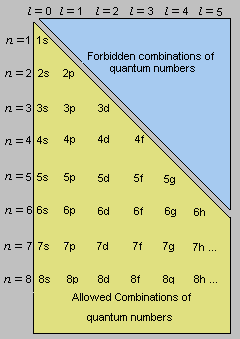The third rule limiting allowed combinations of the n, l, and m quantum numbers has an important consequence. It forces the number of subshells in a shell to be equal to the principal quantum number for the shell. The n = 3 shell, for example, contains three subshells: the 3s, 3p, and 3d orbitals.Possible Combinations of Quantum Numbers

There is only one orbital in the n = 1 shell because there is only one way in which a sphere can be oriented in space. The only allowed combination of quantum numbers for which n = 1 is the following.

 n l m 1 0 0 1s

There are four orbitals in the n = 2 shell.

 n l m 2 0 0 2s
 2 1 -12 1 0 2p 2 1 1

There is only one orbital in the 2s subshell. But, there are three orbitals in the 2p subshell because there are three directions in which a p orbital can point. One of these orbitals is oriented along the X axis, another along the Y axis, and the third along the Z axis of a coordinate system, as shown in the figure below. These orbitals are therefore known as the 2px, 2py, and 2pz orbitals.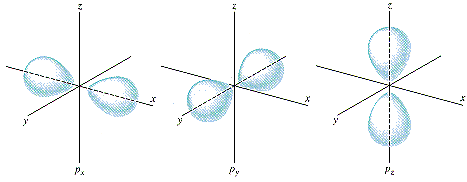There are nine orbitals in the n = 3 shell.

 n l m 3 0 0 3s 3 1 -13 1 0 3p 3 1 1 3 2 -23 2 -1 3d 3 2 0 3 2 1 3 2 2

There is one orbital in the 3s subshell and three orbitals in the 3p subshell. The n = 3 shell, however, also includes 3d orbitals.

The five different orientations of orbitals in the 3d subshell are shown in the figure below. One of these orbitals lies in the XY plane of an XYZ coordinate system and is called the 3dxy orbital. The 3dxz and 3dyz orbitals have the same shape, but they lie between the axes of the coordinate system in the XZ and YZ planes. The fourth orbital in this subshell lies along the X and Y axes and is called the 3dx2-y2 orbital. Most of the space occupied by the fifth orbital lies along the Z axis and this orbital is called the 3dz2 orbital.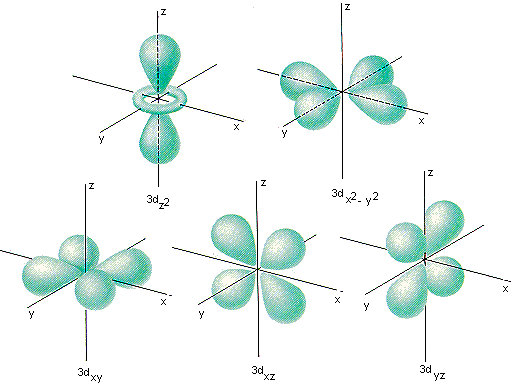The number of orbitals in a shell is the square of the principal quantum number: 12 = 1, 22 = 4, 32 = 9. There is one orbital in an s subshell (l = 0), three orbitals in a p subshell (l = 1), and five orbitals in a d subshell (l = 2). The number of orbitals in a subshell is therefore 2(l) + 1.

Before we can use these orbitals we need to know the number of electrons that can occupy an orbital and how they can be distinguished from one another. Experimental evidence suggests that an orbital can hold no more than two electrons.

To distinguish between the two electrons in an orbital, we need a fourth quantum number. This is called the spin quantum number (s) because electrons behave as if they were spinning in either a clockwise or counterclockwise fashion. One of the electrons in an orbital is arbitrarily assigned an s quantum number of +1/2, the other is assigned an s quantum number of -1/2. Thus, it takes three quantum numbers to define an orbital but four quantum numbers to identify one of the electrons that can occupy the orbital.

The allowed combinations of n, l, and m quantum numbers for the first four shells are given in the table below. For each of these orbitals, there are two allowed values of the spin quantum number, s.Summary of Allowed Combinations of Quantum Numbers

 n l m Subshell Notation Number of Orbitals in the Subshell Number of Electrons Needed to Fill Subshell Total Number of Electrons in Subshell ¯¯¯¯¯¯¯¯¯¯¯¯¯¯¯¯¯¯¯¯¯¯¯¯¯¯¯¯¯¯¯¯¯¯¯¯¯¯¯¯¯¯¯¯¯¯¯¯¯¯¯¯¯¯¯¯¯¯¯¯¯¯¯¯ 1 0 0 1s 1 2 2 ¯¯¯¯¯¯¯¯¯¯¯¯¯¯¯¯¯¯¯¯¯¯¯¯¯¯¯¯¯¯¯¯¯¯¯¯¯¯¯¯¯¯¯¯¯¯¯¯¯¯¯¯¯¯¯¯¯¯¯¯¯¯¯¯ 2 0 0 2s 1 2 2 1 1,0,-1 2p 3 6 8 ¯¯¯¯¯¯¯¯¯¯¯¯¯¯¯¯¯¯¯¯¯¯¯¯¯¯¯¯¯¯¯¯¯¯¯¯¯¯¯¯¯¯¯¯¯¯¯¯¯¯¯¯¯¯¯¯¯¯¯¯¯¯¯¯ 3 0 0 3s 1 2 3 1 1,0,-1 3p 3 6 3 2 2,1,0,-1,-2 3d 5 10 18 ¯¯¯¯¯¯¯¯¯¯¯¯¯¯¯¯¯¯¯¯¯¯¯¯¯¯¯¯¯¯¯¯¯¯¯¯¯¯¯¯¯¯¯¯¯¯¯¯¯¯¯¯¯¯¯¯¯¯¯¯¯¯¯¯ 4 0 0 4s 1 2 4 1 1,0,-1 4p 3 6 4 2 2,1,0,-1,-2 4d 5 10 4 3 3,2,1,0,-1,-2,-3 4f 7 14 32The Relative Energies of Atomic Orbitals

Because of the force of attraction between objects of opposite charge, the most important factor influencing the energy of an orbital is its size and therefore the value of the principal quantum number, n. For an atom that contains only one electron, there is no difference between the energies of the different subshells within a shell. The 3s, 3p, and 3d orbitals, for example, have the same energy in a hydrogen atom. The Bohr model, which specified the energies of orbits in terms of nothing more than the distance between the electron and the nucleus, therefore works for this atom.

The hydrogen atom is unusual, however. As soon as an atom contains more than one electron, the different subshells no longer have the same energy. Within a given shell, the s orbitals always have the lowest energy. The energy of the subshells gradually becomes larger as the value of the angular quantum number becomes larger.

Relative energies: s < p < d < f

As a result, two factors control the energy of an orbital for most atoms: the size of the orbital and its shape, as shown in the figure below.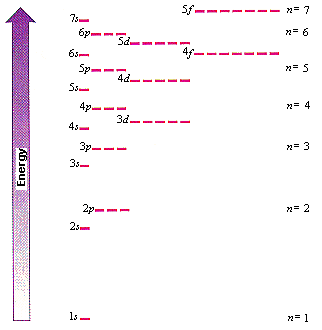A very simple device can be constructed to estimate the relative energies of atomic orbitals. The allowed combinations of the n and l quantum numbers are organized in a table, as shown in the figure below and arrows are drawn at 45 degree angles pointing toward the bottom left corner of the table.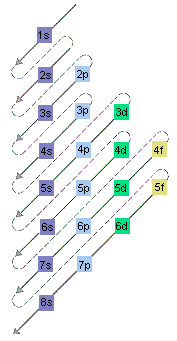The order of increasing energy of the orbitals is then read off by following these arrows, starting at the top of the first line and then proceeding on to the second, third, fourth lines, and so on. This diagram predicts the following order of increasing energy for atomic orbitals.

1s < 2s < 2p < 3s < 3p <4s < 3d <4p < 5s < 4d < 5p < 6s < 4f < 5d < 6p < 7s < 5f < 6d < 7p < 8s ...Electron Configurations, the Aufbau Principle, Degenerate Orbitals, and Hund's Rule

The electron configuration of an atom describes the orbitals occupied by electrons on the atom. The basis of this prediction is a rule known as the aufbau principle, which assumes that electrons are added to an atom, one at a time, starting with the lowest energy orbital, until all of the electrons have been placed in an appropriate orbital.

A hydrogen atom (Z = 1) has only one electron, which goes into the lowest energy orbital, the 1s orbital. This is indicated by writing a superscript "1" after the symbol for the orbital.

H (Z = 1): 1s1

The next element has two electrons and the second electron fills the 1s orbital because there are only two possible values for the spin quantum number used to distinguish between the electrons in an orbital.

He (Z = 2): 1s2

The third electron goes into the next orbital in the energy diagram, the 2s orbital.

Li (Z = 3): 1s2 2s1

The fourth electron fills this orbital.

Be (Z = 4): 1s2 2s2

After the 1s and 2s orbitals have been filled, the next lowest energy orbitals are the three 2p orbitals. The fifth electron therefore goes into one of these orbitals.

B (Z = 5): 1s2 2s2 2p1

When the time comes to add a sixth electron, the electron configuration is obvious.

C (Z = 6): 1s2 2s2 2p2

However, there are three orbitals in the 2p subshell. Does the second electron go into the same orbital as the first, or does it go into one of the other orbitals in this subshell?

To answer this, we need to understand the concept of degenerate orbitals. By definition, orbitals are degenerate when they have the same energy. The energy of an orbital depends on both its size and its shape because the electron spends more of its time further from the nucleus of the atom as the orbital becomes larger or the shape becomes more complex. In an isolated atom, however, the energy of an orbital doesn't depend on the direction in which it points in space. Orbitals that differ only in their orientation in space, such as the 2px, 2py, and 2pz orbitals, are therefore degenerate.

Electrons fill degenerate orbitals according to rules first stated by Friedrich Hund. Hund's rules can be summarized as follows.

• One electron is added to each of the degenerate orbitals in a subshell before two electrons are added to any orbital in the subshell.
• Electrons are added to a subshell with the same value of the spin quantum number until each orbital in the subshell has at least one electron.

When the time comes to place two electrons into the 2p subshell we put one electron into each of two of these orbitals. (The choice between the 2px, 2py, and 2pz orbitals is purely arbitrary.)

C (Z = 6): 1s2 2s2 2px1 2py1

The fact that both of the electrons in the 2p subshell have the same spin quantum number can be shown by representing an electron for which s = +1/2 with an

arrow pointing up and an electron for which s = -1/2 with an arrow pointing down.

The electrons in the 2p orbitals on carbon can therefore be represented as follows.When we get to N (Z = 7), we have to put one electron into each of the three degenerate 2p orbitals.

 N (Z = 7): 1s2 2s2 2p3Because each orbital in this subshell now contains one electron, the next electron added to the subshell must have the opposite spin quantum number, thereby filling one of the 2p orbitals.

 O (Z = 8): 1s2 2s2 2p4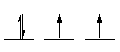The ninth electron fills a second orbital in this subshell.

 F (Z = 9): 1s2 2s2 2p5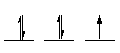The tenth electron completes the 2p subshell.

 Ne (Z = 10): 1s2 2s2 2p6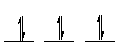There is something unusually stable about atoms, such as He and Ne, that have electron configurations with filled shells of orbitals. By convention, we therefore write abbreviated electron configurations in terms of the number of electrons beyond the previous element with a filled-shell electron configuration. Electron configurations of the next two elements in the periodic table, for example, could be written as follows.

Na (Z = 11): [Ne] 3s1

Mg (Z = 12): [Ne] 3s2

 Practice Problem 8:Predict the electron configuration for a neutral tin atom (Sn, Z = 50). Click here to check your answer to Practice Problem 8

The aufbau process can be used to predict the electron configuration for an element. The actual configuration used by the element has to be determined experimentally. The experimentally determined electron configurations for the elements in the first four rows of the periodic table are given in the table in the following section.

 Learning ActivityThe Electron Configurations of the Elements

(1st, 2nd, 3rd, and 4th Row Elements)

 Atomic Number Symbol Electron Configuration ¯¯¯¯¯¯¯¯¯¯¯¯¯¯¯¯¯¯¯¯¯¯¯¯¯¯¯¯¯¯¯¯¯¯¯¯¯¯¯¯¯¯¯¯¯¯¯¯¯¯¯¯¯¯¯¯¯¯¯¯¯¯¯¯ 1 H 1s1 2 He 1s2 = [He] 3 Li [He] 2s1 4 Be [He] 2s2 5 B [He] 2s2 2p1 6 C [He] 2s2 2p2 7 N [He] 2s2 2p3 8 O [He] 2s2 2p4 9 F [He] 2s2 2p5 10 Ne [He] 2s2 2p6 = [Ne] 11 Na [Ne] 3s1 12 Mg [Ne] 3s2 13 Al [Ne] 3s2 3p1 14 Si [Ne] 3s2 3p2 15 P [Ne] 3s2 3p3 16 S [Ne] 3s2 3p4 17 Cl [Ne] 3s2 3p5 18 Ar [Ne] 3s2 3p6 = [Ar] 19 K [Ar] 4s1 20 Ca [Ar] 4s2 21 Sc [Ar] 4s2 3d1 22 Ti [Ar] 4s2 3d2 23 V [Ar] 4s2 3d3 24 Cr [Ar] 4s1 3d5 25 Mn [Ar] 4s2 3d5 26 Fe [Ar] 4s2 3d6 27 Co [Ar] 4s2 3d7 28 Ni [Ar] 4s2 3d8 29 Cu [Ar] 4s1 3d10 30 Zn [Ar] 4s2 3d10 31 Ga [Ar] 4s2 3d10 4p1 32 Ge [Ar] 4s2 3d10 4p2 33 As [Ar] 4s2 3d10 4p3 34 Se [Ar] 4s2 3d10 4p4 35 Br [Ar] 4s2 3d10 4p5 36 Kr [Ar] 4s2 3d10 4p6 = [Kr]Exceptions to Predicted Electron Configurations

There are several patterns in the electron configurations listed in the table in the previous section. One of the most striking is the remarkable level of agreement between these configurations and the configurations we would predict. There are only two exceptions among the first 40 elements: chromium and copper.

Strict adherence to the rules of the aufbau process would predict the following electron configurations for chromium and copper.

 predicted electron configurations: Cr (Z = 24): [Ar] 4s2 3d4 Cu (Z = 29): [Ar] 4s2 3d9

The experimentally determined electron configurations for these elements are slightly different.

 actual electron configurations: Cr (Z = 24): [Ar] 4s1 3d5 Cu (Z = 29): [Ar] 4s1 3d10

In each case, one electron has been transferred from the 4s orbital to a 3d orbital, even though the 3d orbitals are supposed to be at a higher level than the 4s orbital.

Once we get beyond atomic number 40, the difference between the energies of adjacent orbitals is small enough that it becomes much easier to transfer an electron from one orbital to another. Most of the exceptions to the electron configuration predicted from the aufbau diagram shown earlier therefore occur among elements with atomic numbers larger than 40. Although it is tempting to focus attention on the handful of elements that have electron configurations that differ from those predicted with the aufbau diagram, the amazing thing is that this simple diagram works for so many elements.Electron Configurations and the Periodic Table

When electron configuration data are arranged so that we can compare elements in one of the horizontal rows of the periodic table, we find that these rows typically correspond to the filling of a shell of orbitals. The second row, for example, contains elements in which the orbitals in the n = 2 shell are filled.

 Li (Z = 3): [He] 2s1 Be (Z = 4): [He] 2s2 B (Z = 5): [He] 2s2 2p1 C (Z = 6): [He] 2s2 2p2 N (Z = 7): [He] 2s2 2p3 O (Z = 8): [He] 2s2 2p4 F (Z = 9): [He] 2s2 2p5 Ne (Z = 10): [He] 2s2 2p6

There is an obvious pattern within the vertical columns, or groups, of the periodic table as well. The elements in a group have similar configurations for their outermost electrons. This relationship can be seen by looking at the electron configurations of elements in columns on either side of the periodic table.

 Group IA Group VIIA H 1s1 Li [He] 2s1 F [He] 2s2 2p5 Na [Ne] 3s1 Cl [Ne] 3s2 3p5 K [Ar] 4s1 Br [Ar] 4s2 3d10 4p5 Rb [Kr] 5s1 I [Kr] 5s2 4d10 5p5 Cs [Xe] 6s1 At [Xe] 6s2 4f14 5d10 6p5

The figure below shows the relationship between the periodic table and the orbitals being filled during the aufbau process. The two columns on the left side of the periodic table correspond to the filling of an s orbital. The next 10 columns include elements in which the five orbitals in a d subshell are filled. The six columns on the right represent the filling of the three orbitals in a p subshell. Finally, the 14 columns at the bottom of the table correspond to the filling of the seven orbitals in an f subshell.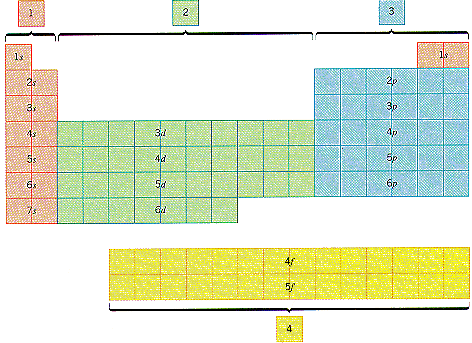Practice Problem 9:Predict the electron configuration for calcium (Z = 20) and zinc (Z = 30) from their positions in the periodic table. Click here to check your answer to Practice Problem 9